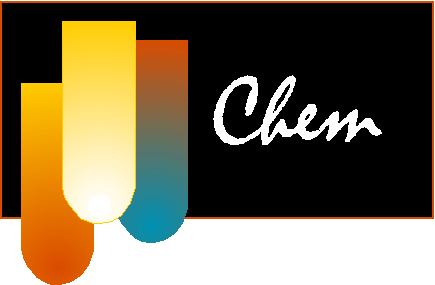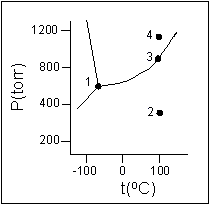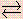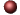Liquids and SolidsExamples ofMultiple Choice Questions

1.
What type of intermolecular forces are due to the attraction between temporary dipoles and their induced temporary dipoles?
(a) metallic bond
(b) London dispersion
(c) hydrogen bond
(d) ionic bond
(e) covalent bond

2.
What type of interparticle forces holds liquid N2 together?
(a) ionic bonding
(b) London forces
(c) hydrogen bonding
(d) dipole-dipole interaction
(e) covalent bonding

3.
Which response includes only those compounds that can exhibit hydrogen bonding?
CH4, AsH3, CH3NH2, H2Te, HF
(a) AsH3, H2Te
(b) AsH3, CH3NH2
(c) CH4, AsH3, H2Te
(d) CH3NH2, HF
(e) HF, H2Te

4.
Which of the following boils at the highest temperature?
(a) CH4
(b) C2H6
(c) C3H8
(d) C4H10
(e) C5H12

5.
Which probably has the lowest boiling point at 1.00 atm?
(a) HF
(b) HCl
(c) HBr
(d) HI
(e) H2SO4

6.
The normal boiling point of a liquid is
(a) the temperature at which the vapor pressure equals 760 torr.
(b) the temperature above which the substance cannot exist as a liquid regardless of the pressure.
(c) the temperature at which the gas molecules have more kinetic energy than the molecules in the liquid.
(d) the only temperature at which there can be equilibrium between liquid and gas.
(e) the temperature at which the liquid will usually boil.

7.
Which of the following changes would increase the vapor pressure of a liquid?
1. an increase in temperature
2. an increase in the intermolecular forces in the liquid
3. an increase in the size of the open vessel containing the liquid

(a) 1 and 2 only
(b) 1 and 3 only
(c) 1 only
(d) 2 only
(e) 3 only

8.
For water (m.p. 0oC, b.p. 100oC)
Heat of fusion = 333 J/g @ 0oC
Heat of vaporization = 2260 J/g @ 100oC
Specific Heat (solid) = 2.09 J/goC
Specific Heat (liquid) = 4.18 J/goC
Specific Heat (gas) = 2.03 J/goC

Calculate the amount of heat (in kJ) that must be absorbed to convert 108 g of ice at 0oC to water at 70oC.

(a) 77
(b) 68
(c) 64
(d) 57
(e) 50

9.
For mercury (m.p. -39oC, b.p. 357oC)
Heat of fusion = 11.6 J/g @ -39oC
Heat of vaporization = 292 J/g @ 357oC
Specific Heat (solid) = 0.141 J/goC
Specific Heat (liquid) = 0.138 J/goC
Specific Heat (gas) = 0.104 J/goC

Calculate the amount of heat that must be released to convert 20.0 g of mercury vapor at 387 oC to liquid mercury at 307oC (in kJ).

(a) 61.9
(b) 6.56
(c) 6.04
(d) 5.69
(e) 5.10

10.
Which of the following phase changes is(are) endothermic?
 1. melting 3. sublimation 5. deposition 2. vaporization 4. condensation 6. freezing
(a) 1, 2, and 3
(b) 4, 5, and 6
(c) 1 and 2 only
(d) 4 and 6 only
(e) some other combination

11.
According to the phase diagram given for Compound Y, what description is correct?(a) At the temperature and pressure at point 4, Y(g) will spontaneously convert to Y(l).
(b) At 0oC and 1200 torr, Y exists as a solid.
(c) At the pressure and temperature of point 1, Y(s) will spontaneously convert to Y(g) and no Y(l) is possible.
(d) At the pressure and temperature at point 3, Y(s)Y(g).
(e) At the temperature and pressure at point 2, Y(l)Y(g)

12.
Where on a phase diagram can you locate conditions under which only one phase exists?
(a) at an intersection of two lines
(b) at the normal boiling point
(c) at an intersection of three lines
(d) in an area bounded by lines
(e) at the triple point

13.
In any cubic lattic, an atom lying at the corner of a unit cell is shared equally by how many unit cells?
(a) one
(b) two
(c) eight
(d) four
(e) sixteen

14.
Which statement is false?
(a) Molecular solids generally have lower melting points than covalent solids.
(b) Metallic solids exhibit a wide range of melting points because metallic bonds cover a wide range of bond strength.
(c) The metallic solid can be viewed as positive ions closely packed in a sea of valence electrons.
(d) Most molecular solids melt at lower temperatures than metallic solids.
(e) The interactions among the molecules in molecular solids are generally stronger than those among the particles that define either covalent or ionic crystal lattices.

15.
Which one of the following classifications is incorrect?
(a) H2O(s), molecular solid
(b) C4H10(s), molecular solid
(c) KF(s), ionic solid
(d) SiC(s), covalent solid
(e) S(s), metallic solid

16.
Which of the following compounds would be expected to have the highest melting point?
(a) BaF2
(b) BaCl2
(c) BaBr2
(d) BaI2
(e) H2O

17.
Which one of the following substances can be melted without breaking chemical bonds?
(a) sodium sulfate
(b) zinc chloride
(c) sulfur dioxide
(d) silicon dioxide
(e) diamond

1. (b) 2. (b) 3. (d) 4. (e) 5. (b) 6. (a) 7. (c) 8. (b) 9. (c) 10. (a) 11. (a) 12. (d) 13. (c) 14. (e) 15. (e) 16. (a) 17. (c)Click here to return to the top.Choose your next chapter:
Fundamentals of Chemistry | Chemical Formulas & Composition Stoichiometry | Chemical Equations & Rxn Stoichiometry | Types of Chemical Reactions |
| Atomic Structure | Chemical Periodicity | Chemical Bonding | Molecular Structure/Covalent Bonding Theories| Molecular Orbital Theory |
| Acids/Bases/Salts - Theory & Rxns | Acids/Bases/Salts - Calculations (including balancing redox rxns) | Gases | Solids & Liquids | Solutions |
| Thermodynamics | Kinetics | Equilibrium | Aqueous Equilibrium - Acids/Bases/Salts | Aqueous Equilibrium - Buffers & Titrations |
| Aqueous Equilibrium - Slightly Soluble Salts | Electrochemistry | Metallurgy | Metal Properties & Rxns | Nonmetals & Metalloids |
| Coordination Compounds | Nuclear Chemistry | Organic Chem - Formulas/Names/Properties | Organic Chem - Shapes/Rxns/Biopolymers |To report any corrections, please e-mail Dr. Wendy Keeney-Kennicutt.